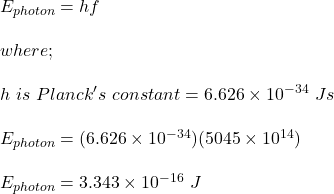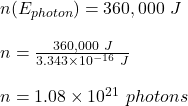g to generate electricity, solar panels typically absorb visible light. How many photons of light with a frequency of 5045×10 14 hz does a s

Question

g to generate electricity, solar panels typically absorb visible light. How many photons of light with a frequency of 5045×10 14 hz does a solar panel absorb to create 360 kj

in progress 0
6 months 2021-07-15T20:20:37+00:00 1 Answers 6 views 0

the number of photons absorbed by the solar panel is 1.08 x 10²¹

Explanation:

Given;

frequency of each photon absorbed, f = 5045 x 10¹⁴ Hz

energy to be created by the solar panel, E = 360 kJ = 360,000 J

The energy of each photon absorbed is calculated as;let the number of photons absorbed = nTherefore, the number of photons absorbed by the solar panel is 1.08 x 10²¹